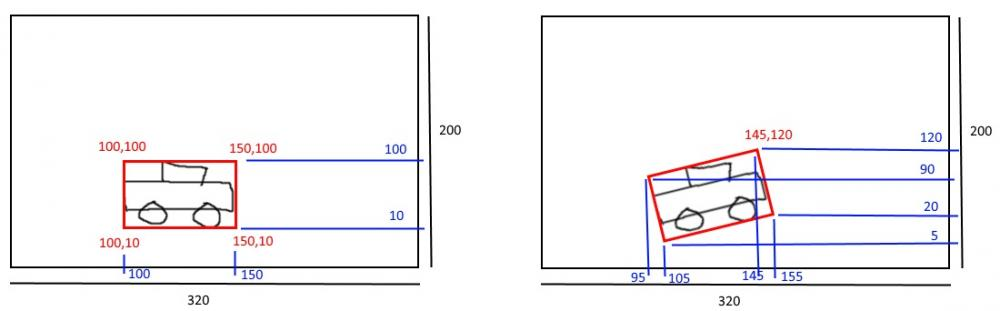# Relative values of vertices of polygon in sprite

## Recommended Posts

Hi.. I would like to know if theres a way of getting the values of vertices in a p2 polygon loaded to sprite (in relative values to the screen)

In example, I have a car sprite loaded with a rectangular polygon:

var car = game.add.sprite(x, y, sprite);
game.physics.p2.enable(car, true);

Later, I rotate the sprite and the vertices "changed" (the X and Y values shown in the image are aproximate)

So, what i want to know it's how to get the vertices {(100,100),(150, 100),(150,10),(100,10)}, or the rotated ones relative to the 320x200 screen.

When I debug the game, and navigate with chrome, the hierarchy Sprite.body.data.shape.vertices[] are

vertices:Array(4)
0:Float32Array(2) [-0.5, -0.75]
1:Float32Array(2) [0.5, -0.75]
2:Float32Array(2) [0.5, 0.75]
3:Float32Array(2) [-0.5, 0.75]

And after rotating the car, the vertices are the same.

Thank you in advance, and sorry for my english!##### Share on other sites

Vertices of a p2 polygon are relative to the center of the polygon. Supposed  you wanted a 2x2 square, the vertices would be [ [-1, -1], [1, -1], [1, 1], [-1, 1] ] (CCW direction).

When your sprite is at [100, 100], the first vertex would be at 100 + game.p2.mpx(-1) = 80. Where, game.p2.mpx() converts meters(p2 units) to px(Phaser units). If you scale the sprite, you need to take that into account as well.

If you rotate a sprite and the p2 vertices stay the same, you can calculate them like so:

``````
function myFunction(){
//get rotated vertex in screen coordinates.
let p2x = sprite.x + game.p2.mpx(vertices);
let p2y = sprite.y + game.p2.mpx(vertices);
let transformedCoordinates = transformed(p2x, p2y, sprite.x, sprite.y, sprite.rotation);

console.log("x0: "+transformedCoordinates+ "y0: "+transformedCoordinates);
}

function transformed(x: number, y: number, cx: number, cy: number, slope: number) {
let nx = x - cx;
let ny = y - cy;
let tx = nx * Math.cos(slope) - ny * Math.sin(slope);
let ty = nx * Math.sin(slope) + ny * Math.cos(slope);
return [tx + cx, ty + cy]
}``````

##### Share on other sites

Dear gauravD, I finally fixit doing something very similar of your code:

``````	function getCollisionRectangle(sprite) {
var shape = sprite.body.data.shapes;
var shape = new p2.Box({ width: game.physics.p2.mpx(shape.width), height: game.physics.p2.mpx(shape.height)});
var points = [];
console.log('------------');
for(var i = 0; i < 4; ++i) {
var vrot = p2.vec2.create();
var v = shape.vertices[i];
var rotation = sprite.body.rotation;
p2.vec2.rotate(vrot, v, rotation);

points.push(vrot);
console.log('vrot: ' + vrot);
}

return points;
}``````

Thank you!